# High School Math : How to find an angle in a polygon

## Example Questions

### Example Question #1 : How To Find An Angle In A Polygon

What is the magnitude of the interior angle of a regular nonagon?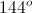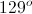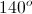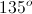Explanation:

The equation to calculate the magnitude of an interior angle is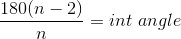, whereis equal to the number of sides.

For our question,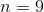.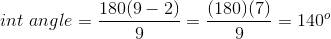### Example Question #2 : How To Find An Angle In A Polygon

What is the interior angle measure of any regular heptagon?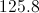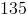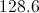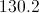Explanation:

To find the angle of any regular polygon you find the number of sides,. In this example,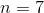.

You then subtract 2 from the number of sides yielding 5.

Take 5 and multiply it by 180 degrees to yield the total number of degrees in the regular heptagon.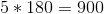Then to find one individual angle we divide 900 by the total number of angles, 7.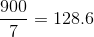The answer is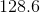.

### Example Question #3 : How To Find An Angle In A Polygon

A regular polygon withsides has exterior angles that measure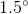each. How many sides does the polygon have?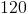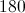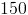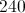This figure cannot exist.Explanation:

The sum of the exterior angles of any polygon, one per vertex, is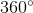. As each angle measures, just divide 360 by 1.5 to get the number of angles.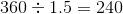### Example Question #4 : How To Find An Angle In A Polygon

What is the interior angle measure of any regular nonagon?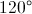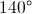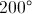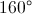Explanation:

To find the angle of any regular polygon you find the number of sides, which in this example is.

You then subtractfrom the number of sides yielding.

Takeand multiply it bydegrees to yield a total number of degrees in the regular nonagon.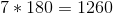Then to find one individual angle we divide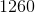by the total number of angles.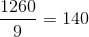The answer is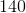.

### Example Question #5 : How To Find An Angle In A Polygon

What is the measure of one exterior angle of a regular seventeen-sided polygon (nearest tenth of a degree)?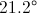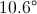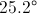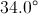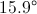Explanation:

The sum of the measures of the exterior angles of any polygon, one per vertex, is. In a regular polygon, all of these angles are congruent, so divide 360 by 17 to get the measure of one exterior angle: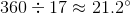### Example Question #6 : How To Find An Angle In A Polygon

What is the measure of one exterior angle of a regular twenty-three-sided polygon (nearest tenth of a degree)?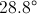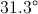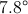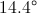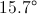Explanation:

The sum of the measures of the exterior angles of any polygon, one per vertex, is. In a regular polygon, all of these angles are congruent, so divide 360 by 23 to get the measure of one exterior angle: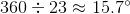### Example Question #7 : How To Find An Angle In A Polygon

What is the measure of one interior angle of a regular twenty-three-sided polygon (nearest tenth of a degree)?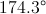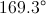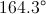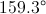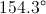Explanation:

The measure of each interior angle of a regular polygon withsides is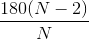. We can substitute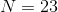to obtain the angle measure: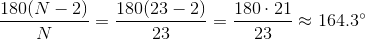### Example Question #8 : How To Find An Angle In A Polygon

A regular polygon has interior angles which measure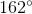each. How many sides does the polygon have?Explanation:

The easiest way to answer this is to note that, since an interior angle and an exterior angle form a linear pair - and thus, a supplementary pair - each exterior angle would have measure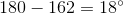. Since 360 divided by the number of sides of a regular polygon is equal to the measure of one of its exterior angles, we are seekingsuch that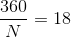Solve for: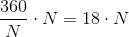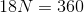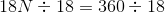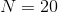The polygon has 20 sides.